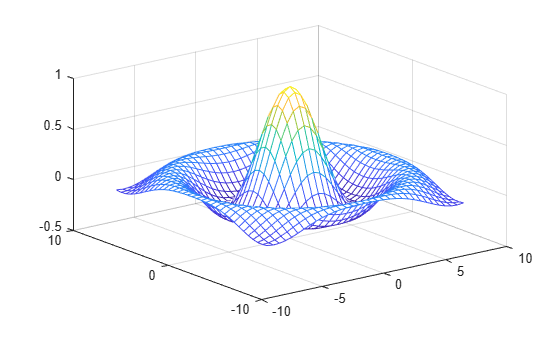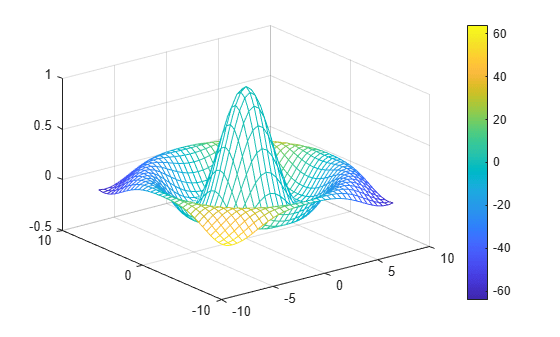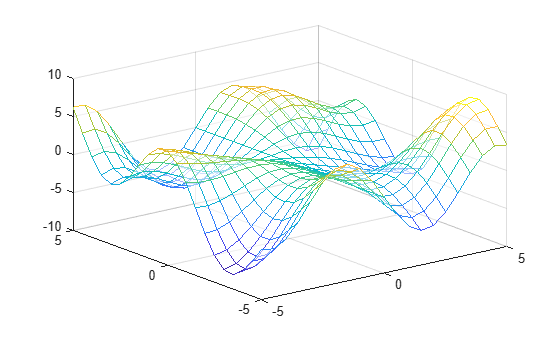Documentation

### This is machine translation

Mouseover text to see original. Click the button below to return to the English version of the page.

# mesh## Syntax

```mesh(X,Y,Z) mesh(Z) mesh(...,C) mesh(...,'PropertyName',PropertyValue,...) mesh(axes_handles,...) s = mesh(...) ```

## Description

`mesh(X,Y,Z)` draws a wireframe mesh with color determined by `Z`, so color is proportional to surface height. If `X` and `Y` are vectors, `length(X) = n` and ```length(Y) = m```, where `[m,n] = size(Z)`. In this case, (X(j), Y(i), Z(i,j)) are the intersections of the wireframe grid lines; `X` and `Y` correspond to the columns and rows of `Z`, respectively. If `X` and `Y` are matrices, (X(i,j), Y(i,j), Z(i,j)) are the intersections of the wireframe grid lines. The values in `X`, `Y`, or `Z` can be numeric, datetime, duration, or categorical values.

`mesh(Z)` draws a wireframe mesh using `X = 1:n` and `Y = 1:m`, where `[m,n] = size(Z)`. The height, `Z`, is a single-valued function defined over a rectangular grid. Color is proportional to surface height. The values in `Z` can be numeric, datetime, duration, or categorical values.

`mesh(...,C)` draws a wireframe mesh with color determined by matrix `C`. MATLAB® performs a linear transformation on the data in `C` to obtain colors from the current colormap. If `X`, `Y`, and `Z` are matrices, they must be the same size as `C`.

`mesh(...,'PropertyName',PropertyValue,...)` sets the value of the specified surface property. Multiple property values can be set with a single statement.

`mesh(axes_handles,...)` plots into the axes with handle `axes_handle` instead of the current axes (`gca`).

`s = mesh(...)` returns a Surface Properties object.

## Examples

collapse all

Create a mesh plot of the sinc function, $z=\mathrm{sin}\left(r\right)/r$.

```[X,Y] = meshgrid(-8:.5:8); R = sqrt(X.^2 + Y.^2) + eps; Z = sin(R)./R; mesh(X,Y,Z)```Specify a color matrix for a mesh plot.

```[X,Y] = meshgrid(-8:.5:8); R = sqrt(X.^2 + Y.^2) + eps; Z = sin(R)./R; C = gradient(Z); figure mesh(X,Y,Z,C)```Change the lighting and the line width for a mesh plot using `Name,Value` pair arguments.

```[X,Y] = meshgrid(-8:.5:8); R = sqrt(X.^2 + Y.^2) + eps; Z = sin(R)./R; C = del2(Z); figure mesh(X,Y,Z,C,'FaceLighting','gouraud','LineWidth',0.3)```## Tips

`mesh` does not accept complex inputs.

A mesh is drawn as a `Surface` object with the viewpoint specified by `view(3)`. The face color is the same as the background color (to simulate a wireframe with hidden-surface elimination), or `none` when drawing a standard see-through wireframe. The current colormap determines the edge color. The `hidden` command controls the simulation of hidden-surface elimination in the mesh, and the `shading` command controls the shading model.